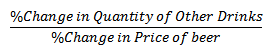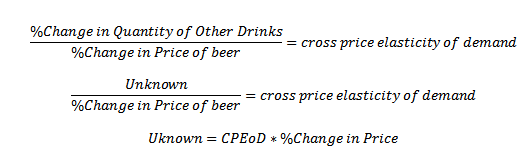Real world example of economics: an application using elasticities - FreeEconHelp.com, Learning Economics... Solved!

## 7/11/11

I was reading an article on yahoo! about tough interview questions.  Most of them are tricky, and to be honest some are obscure such as:

"Estimate the market size of IVF treatment in Sweden." -- For a management consulting position.

Or:

"What is the revenue of an inner city Sydney gym?" -- For an associate consultant position.

Some involved simple math including:

"You have a 10x10x10 rubix cube. You paint the outside. How many cubes have paint on them?" -- For an embedded analyst position.Which is just math, albeit it takes a little while to think about it:
So there are 100 squares on each side, so the first guess is 100*6, but the squares along the edges and corners share paint with other squares so we need to subtract them off.  Eight cubes are corners, which means we subtract 16 (2*8) cubes so they are not double counted (having three colors), and 96 cubes are on the edges (12 edges with 8 cubes each) so another 96 need to be subtracted.  So all in all 600-16-96= 488.  So if you have a 10x10x10 cube, and you paint the outside, then 488 of the little cubes will have paint on them... Whew! not easy, but definitely solvable.

But the question that interested me the most was the one at the bottom of their list which was considered the MOST difficult (which made me laugh).

"A Canadian beer manufacturer notices that when they increase the price of beer, other drinks' consumption goes up. How will you predict the change in quantity bought based on change in price?" -- For an analytics specialist.

This question is just an elasticity question, which anybody who has passed introductory microeconomics should be able to solve.  For this question you just have to know the equation for the cross-price elasticity of demand. In this example, it would be represented by:And since we know that if the price of beer goes up, than other drinks' consumption goes up, then we can determine that the goods substitutes.  To answer the question about predicting the change in quantity bought based on the change in price you would know cross-price elasticity of demand measure, which would be estimated based on prior data you had on drink consumption and beer prices.  Then you could use an example like the equation below to solve for it:Boom, multiply those two together and you will get your estimate.  How easy could this be? And this question was considered the hard one!

For the entire article please go to: